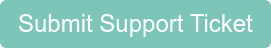## What does excess inventory mean?

#### Excess inventory is the value of inventory on hand beyond what might be needed for typical usage

Excess value is used as an efficiency indicator for money tied up in inventory. A high amount of excess inventory could indicate that there is too much inventory.

To calculate excess inventory, we first must calculate each item's usage. The formula for usage is:

Starting Inventory + Quantity Purchased - Ending Inventory = Usage Quantity

Therefore, in order to produce a usage quantity for each item (and then calculate excess inventory), you must have done at least two inventory sessions in Backbar so we have a starting and ending quantity, and you must also have placed or entered your orders through Backbar. If you do not see any excess inventory or usage data, see Why don't my items show on usage reports for more information.

Once Backbar has usage data for your products, we can calculate excess inventory. Backbar makes the

assumption that you should have enough of a product on hand for a month. This is a generous assumption, as often times you need much less, as you can re-order products from vendors or deal with fluctuations in sales.

Excess inventory = Current Inventory Value - Monthly Average Usage Value

Put another way, we look at what your current quantity on hand is for each item and subtract how much you typically use in a month. This quantity is the excess, more than you need for a month's worth of product. We then multiply the excess quantity by the cost for each item to get the excess value.

#### Still need help?